# 0708-1300/Homework Assignment 10

Announcements go here

## Contents

Read, reread and rereread your notes to this point, and make sure that you really, really really, really really really understand everything in them. Do the same every week!

Also, read section 1 through 6 (to page 183) of chapter IV of Bredon's book three times:

• First time as if you were reading a novel - quickly and without too much attention to detail, just to learn what the main keywords and concepts and goals are.
• Second time like you were studying for an exam on the subject - slowly and not skipping anything, verifying every little detail.
• And then a third time, again at a quicker pace, to remind yourself of the bigger picture all those little details are there to paint.

## Doing

Solve the following problems from Bredon's book, but submit only the solutions of the problems marked with an "S" - problems 1, 2, S3 on pages 176-177, problems S2, 3 on page 182, and problem S5 on page 190. Also, solve and submit the following question:

Problem 6. "Homotopies between maps" define an "ideal" within the category of topological spaces and continuous maps between them: the homotopy relation is an equivalence relation, and if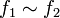$f_1\sim f_2$, then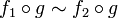$f_1\circ g\sim f_2\circ g$ and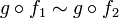$g\circ f_1\sim g\circ f_2$ whenever these compositions make sense. Show that the same is true for the notion "homotopy of chain maps between chain complexes", within the category of chain complexes and chain maps between them.

## Due Date

This assignment is due in class on Thursday March 13, 2008.

## Just for Fun

1. A maximal tree is chosen within the edges of the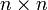$n\times n$ integer lattice. Show that there are two neigboring points on the lattice whose distance from each other, measured only along the tree, is at least$n$.
2. In class we have shown that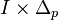$I\times\Delta_p$, that is,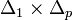$\Delta_1\times\Delta_p$, is a union of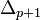$\Delta_{p+1}$'s in a nice and natural way. Can you show that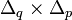$\Delta_q\times\Delta_p$ is a union of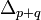$\Delta_{p+q}$'s in a nice and natural way, for general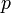$p$ and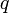$q$?
3. Can you show that the$p$-cube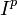$I^p$ is a union of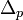$\Delta_p$'s in a nice and natural way?

0708-1300/Just_for_Fun10 is a idea for problem 1 but don't look at it until you have think on it for a while.